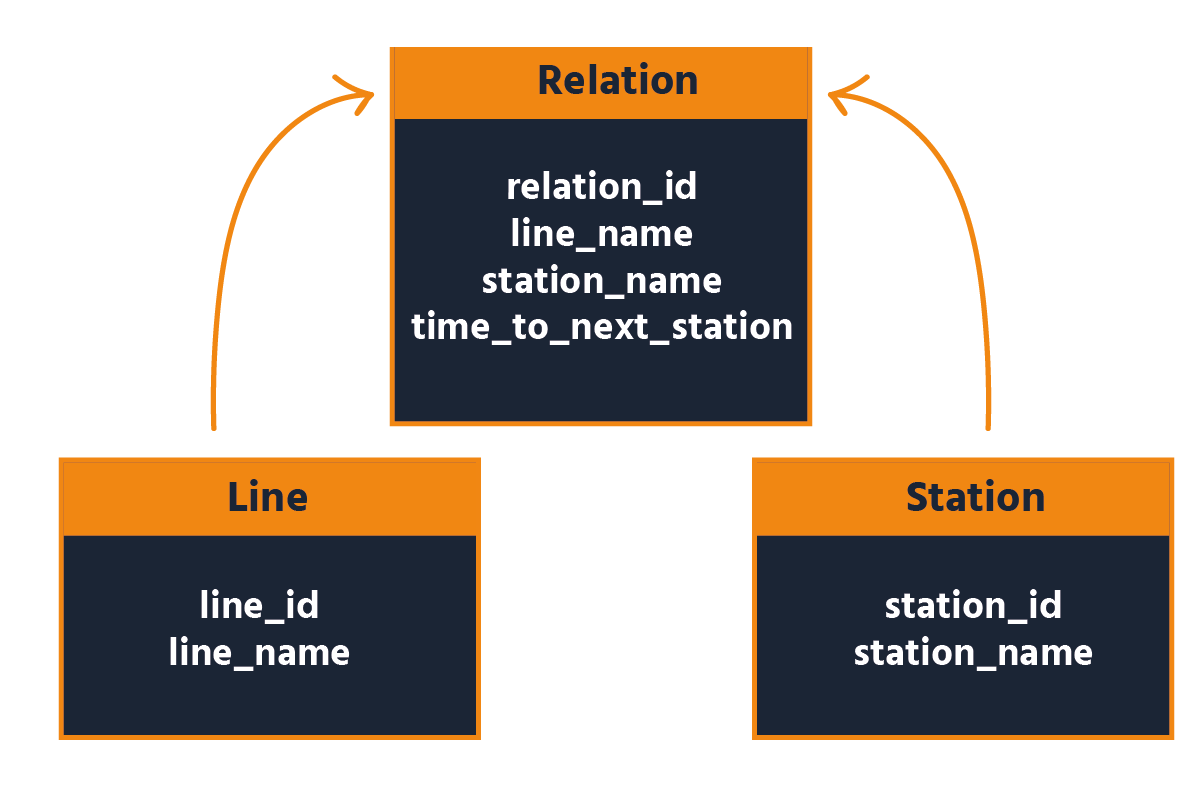Course Content

Intermediate SQL

##GROUP BY

Hi there! Welcome to the Intermediate SQL course! In the first section, we will work with a Monreal metro database containing three tables: `station`, `line` and `relation`. The first table contains the names of metro stations. The second table contains the lines of the Monreal metro. The last table contains the time for each station which you need to get there.

First, we have the `station` table, which contains the names of metro stations. It provides information about individual stationsNext, we have the `line` table, which contains the lines of the Montreal metro. This table helps us understand the various metro lines in Montreal.Lastly, we have the `relation` table, which holds information about the time it takes to reach each station. This table allows us to analyze the connections and travel times between stations.To gain a better understanding of these tables and their relationships, let's examine each one individually. Below, you will find examples that show the structure and content of each table.For instance, you want to get information not about all subway stations separately but for groups of subway stations by lines. Consider an example of finding each subway line's total number of subway stations. To do it, we have to use the `COUNT()` function.

If you run this query, you will get the following result:Analysis: In the above query, we grouped our data by lines using the `GROUP BY` clause, then we counted the numbers of rows in each obtained group using the `COUNT()` function and created a new column for these values named `number_of_station`.

Note

In the example above, we used an alias. This way, we changed and simplified the column name `COUNT(station_name)` to `number_of_station`. We can do the same with the name of any column using this syntax: `column_name AS alias`.

Syntax: To group your data, you just have to use the `GROUP BY` clause in the following way:

It is time to practice!

Using the `relation` table find the longest possible trip (create new column, named `max_time` using `time_to_next_station` and `MAX()` function) for each of the lines (`line_name`).